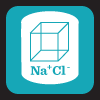Water of Hydration

Last Review: June 2005

Some chemicals may have different degrees of hydration in their molecule. For example, there are two degrees of hydration for citric acid: citric acid anhydrous, which does not contain any water molecule; and citric acid monohydrate, which contains 1 molecule of water. So, practically, if a formula calls for citric acid anhydrous in a solution and the pharmacy has in stock the citric acid monohydrate, the pharmacist must convert the weight of the chemical to account for the water weight.

It is important however to analyze each situation and check if it is appropriate to substitute the chemical in that specific formula. Not all chemicals in formulations may be exchanged since an improper substitution may cause a chemical reaction or even shorten the preparation stability and beyond-use-date.

Example:

To obtain the conversion factor to use the citric acid monohydrate instead of the anhydrous, you can use the following formula:

MW citric acid monohydrate
MW citric acid anhydrous

210.14 = 1.093
192.13

To obtain the conversion factor to use the citric acid anhydrous instead of the monohydrate, you can use the following formula:

MW citric acid anhydrous
MW citric acid monohydrate

192.13 = 0.914
210.14

In order to substitute citric acid anhydrous in a formula for citric acid monohydrate, for each mg of citric acid anhydrous, the pharmacist must weigh 1.093 mg of citric acid monohydrate instead.

In order to substitute citric acid monohydrate in a formula for citric acid anhydrous, for each mg of citric acid monohydrate, the pharmacist must weigh 0.914 mg of citric acid anhydrous instead.

Example Problem:

If a formula calls for 50 mg of citric acid anhydrous and the pharmacy only has citric acid monohydrate, the pharmacist must calculate:

50mg x 1.093 = 54.65mg

Therefore, the pharmacist must weigh out 54.65mg of citric acid monohydrate.